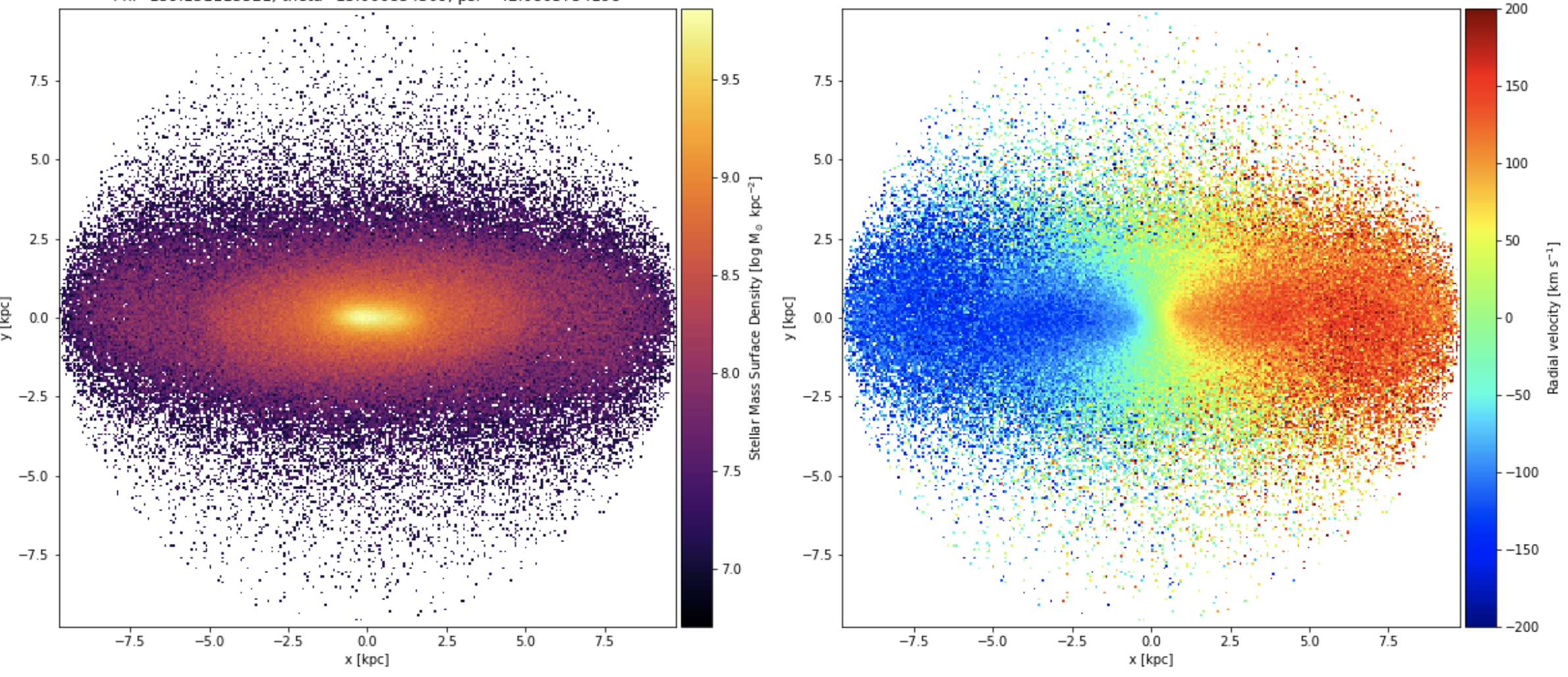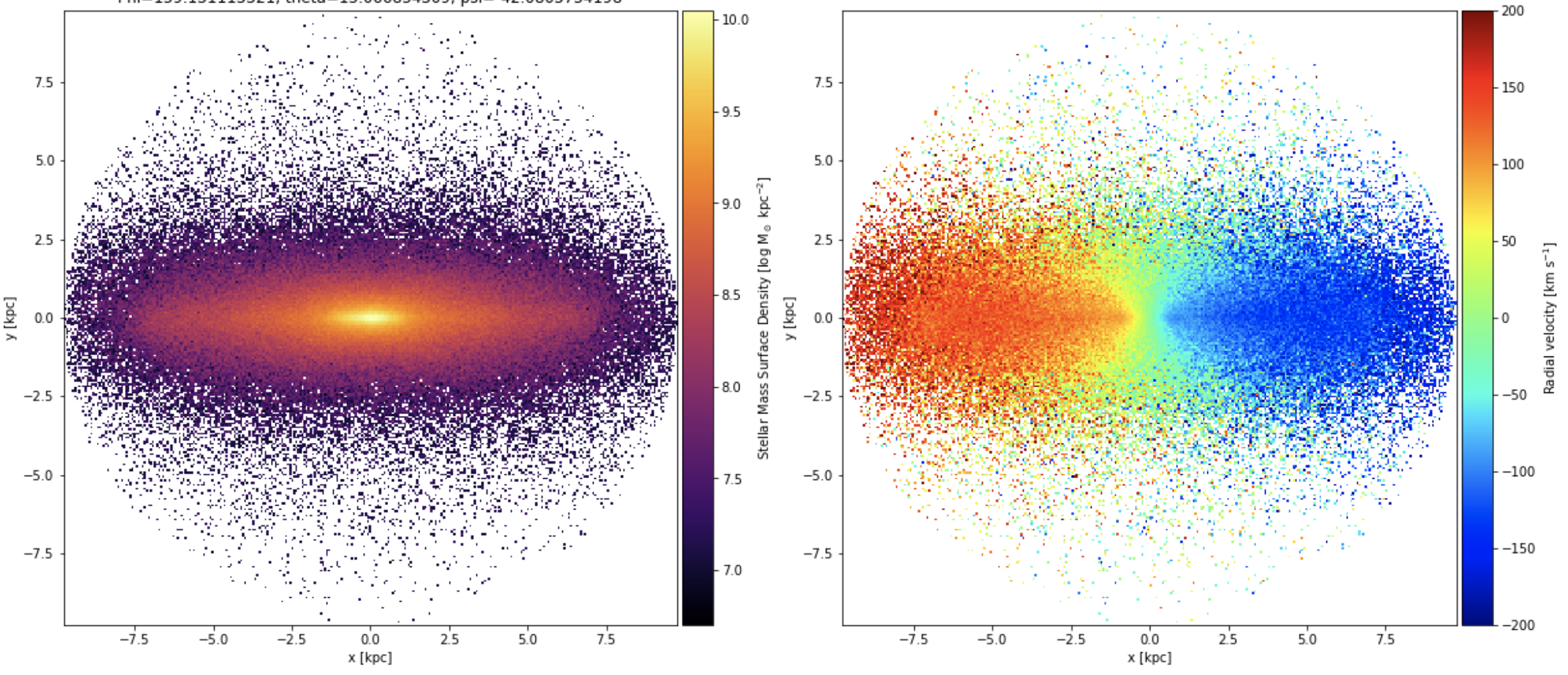### Circularity of edge-on galaxies vs circularity of projected galaxiesPablo Galan de Anta
• 19 Jan

Hi

I need to calculate the necessary angle for a galaxy to become edge-on and, for so, the angle which maximises the angular momentum parallel to the galactic plane. I'm working in projection trying to work in an observational-like approach, and I also have the the machiinery to put the galaxy fully edge-on.

Here it is the galaxy in random projection:And this is the galaxy fully edge-on with maximum angular momentum:My idea is to follow two consecutive steps to get what is the angle to go from the first image to the second on:

1) I align the random projection with my X,Y,Z axes.

2) Rotate the galaxy around the galactic plane (which corresponds to X axis in the images) until I get the maximum value for the circularity (that I can compare with the second image).

However, when I follow this procedure the values I got for the circularity fraction of thin-disc stars (epsilon > 0.7) rotating with different angles around X axes are always much smaller than the circularity fraction of the edge-on view (image 2) and I never get reach the maximum value of the circularity.

Is there any other method to calculate the angle necessary to transform from random inclined galaxy to the fully edge-on view?

PabloDylan Nelson
• 20 Jan

Hi Pablo,

I've not heard before of an approach to do an alignment (i.e. rotate to edge-on) using 2D projections / images / an observationally motivated approach. However, I'm sure that you can get this to work, it seems reasonable.

Typically we would do this in 3D. First, selecting all star-forming gas, or all stars within the stellar half mass radius, or the combination of the two (or any similar choice). Then, two options: (i) calculate the mean angular momentum vector, and then rotate this to align with e.g. the z-axis of your coordinate system. Or (ii) calculate the moment of inertia tensor, from which rotation matrices can be obtained (via diagonalization).Pablo Galan de Anta
• 20 Jan

Hi Dylan,

Yes, I'm actually following the way ii) in order to align the galactic plane of the galaxies with my X-Y-Z axes. In theory, if you align any observed galaxy with your X and Y axes and you perform a rotation around one of this two, from time to time you will maximises the angular momentum and will get the galaxy completely edge-on. The problem comes when the values of the angular momentum highly differ from the values oof the angular momentum in the edge-on view. After centered the coordinates on the minimum potential particle, the code I'm using to do this experiment is the following:

``````    # We need to align the projected galaxy with our X-Y axes
I = np.zeros( (2,2), dtype='float32' )

# Y and Z are the coordinates of the
I[0,0] = np.sum(mass*xx*xx)/np.sum(mass)
I[1,1] = np.sum(mass*yy*yy)/np.sum(mass)
I[0,1] = np.sum(mass*xx*yy)/np.sum(mass)
I[1,0] = I[0,1]

eigen_values, rotation_matrix = np.linalg.eig(I)

# sort ascending the eigen values
sort_inds = np.argsort(eigen_values)
eigen_values = eigen_values[sort_inds]

# permute the eigenvectors into this order, which is the rotation matrix which orients the
# principal axes to the cartesian x,y,z axes
new_matrix = np.matrix( (rotation_matrix[:,sort_inds],
rotation_matrix[:,sort_inds]) )

inc = np.matrix( ((0,1),(1,0)) ) * new_matrix

# Now we rotate the values
xxx = inc[0,0]*xx + inc[0,1]*yy
yyy = inc[1,0]*xx + inc[1,1]*yy

vxx = inc[0,0]*vx + inc[0,1]*vy
vyy = inc[0,0]*vx + inc[0,1]*vy

xx_new,yy_new,zz_new = np.copy(xxx),np.copy(yyy),np.copy(zz)
vv_x,vv_y,vv_z       = np.copy(vxx),np.copy(vyy),np.copy(vz)

# Now we perform a rotation around the X axis
angle = np.radians(57) # Angle for which Jz should be maximum
zzz = np.cos(angle)*zz_new - np.sin(angle)*yy_new
yyy = np.sin(angle)*zz_new + np.cos(angle)*yy_new

vzz = np.cos(angle)*vv_z - np.sin(angle)*vv_y
vyy = np.sin(angle)*vv_z + np.cos(angle)*vv_y

xx_new,yy_new,zz_new = np.copy(xx_new),np.copy(yyy),np.copy(zzz)
vv_x,vv_y,vv_z       = np.copy(vv_x),np.copy(vyy),np.copy(vzz)

# Now we estimate the angular momentum

kpc_to_km = 3.0856e16

# Angular momenta of the three components
jx = kpc_to_km*(yy_new*vv_z - vv_y*zz_new)
jy = kpc_to_km*(vv_x*zz_new - xx_new*vv_z)
jz = kpc_to_km*(xx_new*vv_y - vv_x*yy_new)

jtot = np.sqrt(jx**2 + jy**2 + jz**2)
bind = potential + 0.5*(vv_x**2 + vv_y**2 + vv_z**2)

# Sortened the particles by their binding energy to get the circularity of the particles
idx   = np.argsort(bind)
bind  = bind[idx]; jx_sort = jx[idx]; jy_sort = jy[idx]; jz_sort = jz[idx]; jtot_sort = jtot[idx]
xxx,yyy,zzz = xx_new[idx],yy_new[idx],zz_new[idx]
mass_new    = mass_new[idx];
vvx, vvy, vvz = vv_x[idx], vv_y[idx], vv_z[idx]

circ_neg = np.zeros(len(bind)); circ_pos = np.zeros(len(bind))
for jj in range(len(bind)):
if (jj <= 50) & (jj >= 0):
where = np.arange(0,jj+50)
elif (jj <= len(bind)) & (jj >= len(bind)-50):
where = np.arange(jj-50,len(bind))
else:
where = np.arange(jj-50,jj+50)

# We use the angular momentum parallel to galactic plane to compute the circularity
circ[jj] = jy_sort[jj]/max(jy_sort[where])

CircAbove07Frac = np.sum(mass_new[circ > 0.7])/np.sum(mass_new) # Fraction of 'disc' stars
``````

So if everything goes well, CircAbove07Frac should the same as the one got for the edge-on view, and for so, that would provide me the angle alpha, however, the value I get is very small compare to the edge-on view. Could it be that the galaxy is not perfectly aligned with my XY plane and there's a certain angle? Honestly, I've checked this, but there's something missing that I do not see.Dylan Nelson
• 20 Jan

I see some possible issues, including as you mention that e.g. all disks have some degree of warping, so after an 'optimal' rotation it would not be perfectly aligned with any axis (depending on distance).

Perhaps a way to get very confident in your procedure would be to apply it first to some idealized data (not TNG), i.e. generate some "particles" in a disk where you control exactly their positions, then give it a random rotation, then check that you can recover this rotation with the method.Pablo Galan de Anta
• 20 Jan

Mmmm, yeah, that sounds pretty reasonable. I have a couple of N-body simulated disc galaxies.

Well, maybe instead of trying to get the same circularity value as the one I get for the Edge-on galaxy, i should expect to get the maximum circularity f that projection.